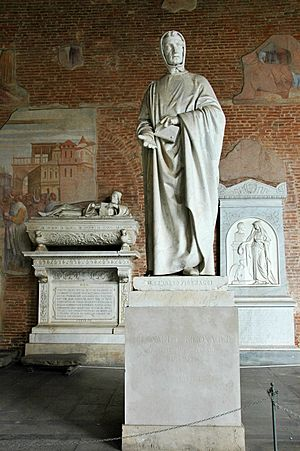# Fibonacci facts for kids

Kids Encyclopedia FactsStatue of Fibonacci by Giovanni Paganucci in the Camposanto di Pisa, 1863

Fibonacci, also known as Leonardo Bonacci, Leonardo Fibonacci and Leonardo of Pisa, lived c. 1170–1250. He was an Italian mathematician. He was thought "the most talented Western mathematician of the Middle Ages".

Fibonacci popularized the Hindu–Arabic numeral system to the Western World. He did this in his composition in 1202 of Liber Abaci (Book of Calculation). He also introduced to Europe the sequence of Fibonacci numbers which he used as an example in Liber Abaci.

## Fibonacci number sequence

Fibonacci is best known for the list of numbers called the Fibonacci Sequence. The list never stops, but it starts this way:

```1,  1,  2,  3,  5,  8,  13,  21,  34,  55,  89, 144, ...
```

In this list, a person can find the next number by adding the last two numbers together.

```1 + 1 = 2
1 + 2 = 3
2 + 3 = 5
3 + 5 = 8
5 + 8 = 13
8 + 13 = 21
13 + 21 = 34
21 + 34 = 55
34 + 55 = 89
55 + 89 = 144
89 + 144 = 233
144 + 233 = 377
233 + 377 = 610
377 + 610 = 987
610 + 987 = 1597
987 + 1597 = 2584
etc...
```

This series is also interesting because the ratio of two adjacent numbers in the series approaches the golden ratio.Fibonacci Facts for Kids. Kiddle Encyclopedia.